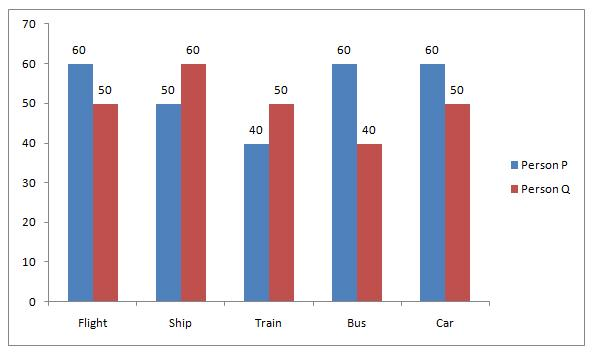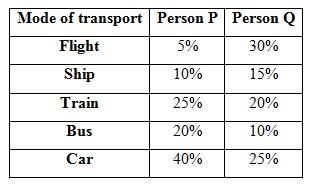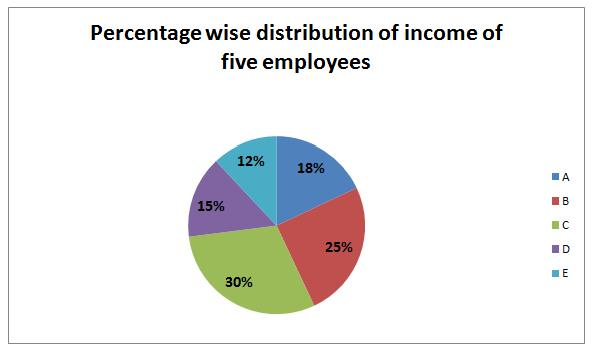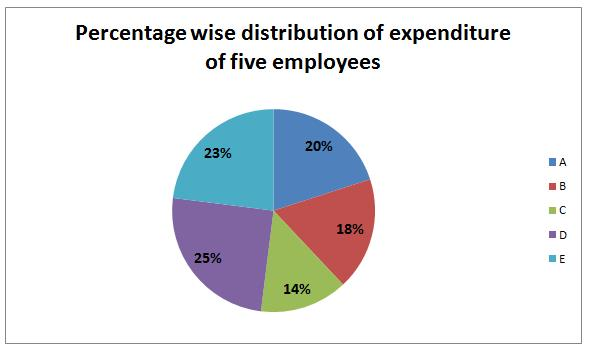# SBI Clerk Mains Quantitative Aptitude (Day-57)

Dear Aspirants, Our IBPS Guide team is providing new series of Quantitative Aptitude Questions for SBI Clerk Mains 2020 so the aspirants can practice it on a daily basis. These questions are framed by our skilled experts after understanding your needs thoroughly. Aspirants can practice these new series questions daily to familiarize with the exact exam pattern and make your preparation effective.

Start Quiz

Ensure Your Ability Before the ExamTake SBI Clerk 2020 Mains Free Mock Test

Data Interpretation

Direction (1-5): Study the following information carefully and answer the questions given below.

The below graph shows the speed (in kmph) of 2 persons while travelling by different mode of transportThe following table shows the percentage of distance travelled by both the persons P and Q by different modes of transport1) If person Q completed his journey by ship in 3.75 hours, then what is the average of the distance travelled by him by train, by bus and by car?

A.200km

B.225km

C.250km

D.275km

E.None of these

2) Suppose, Instead of train, the person P uses Car and if the speed of car is 20% more than the speed of train, then approximately how many hours the person P would save? (Total distance travelled by person P by train is 300km)

A.1 hour

B.1.25 hours

C.1.5 hours

D.2 hours

E.None of these

3) Which of the following statement is definitely true? (Assume that the total distance travelled by person P is 1200km and the total distance travelled by person Q is 1500km)

I: Person P completed his journey by Bus in 6 hours.

II: Person Q completed his journey by Flight in 9 hours.

III: Total time of 12 hours taken by person P to complete his journey by bus and car together.

A.Both I and II

B.Both II and III

C.Only I

D.Only II

E.Only III

4) If person Q completes his journey by Flight in 9 hours, then what is the total distance travelled by person Q in his whole journey?

A.1200km

B.1300km

C.1500km

D.1600km

E.None of these

5) If person P travels 300km by train, then what is the total time taken by person P to travel by Bus and Car together?

A.4 hours

B.8 hours

C.12 hours

D.15 hours

E.None of these

Data Interpretation

Direction (6-10): Study the following information carefully and answer the questions given below.

The following pie charts represents percentage wise distribution of incomes (in Rs.) and expenditure (in Rs.) of five employeesTotal income = Rs.180000Total expenditure = Rs.90000

6) If the income of C is increased by 20% and income of E is increased by 10%, incomes of C and E together is what percent of total income?

A.35.9%

B.39.3%

C.43.1%

D.49.2%

E.None of these

7) If the expenditure of A is increased by 30%, find the percentage decrease in savings?

A.35.4%

B.37.5%

C.43.1%

D.49.2%

E.None of these

8) Find the respective ratio of Income of B and D together and expenditures of C and E together.

A.25: 47

B.80: 37

C.45: 37

D.60: 37

E.None of these

9) Incomes of A and B together is what percentage of savings of B and E together? (approx.)

A.156%

B.170%

C.261%

D.275%

E.290%

10) Find the sum of savings of all the employees?

A.Rs.45000

B.Rs.90000

C.Rs.50000

D.Rs.58000

E.None of these

Distance travelled by Q = Y km

Distance travelled by ship by person Q = 60 * 3.75 = 225 km

Y * 15/100 = 225

=> Y = 1500 km

Distance travelled by train by person Q = 1500 * 20/100 = 300km

Distance travelled by Bus by person Q = 1500 * 10/100 = 150km

Distance travelled by Car by person Q = 1500 * 25/100 = 375km

Average distance = (300 + 150 + 375)/3 = 275km

Speed of car = 120/100 * 40 = 48kmph

Required time = 300/40 – 300/48 = 1.25 hours

From I => Time taken by person P by Bus = (20% of 1200)/60 = 4 hours ≠ 6 hours

This statement is not true.

From II => Time taken by person Q by Flight = (30% of 1500)/50 = 9 hours

This statement is true.

From III => Time taken by person P by bus and car together = (20% of 1200)/60 + (40% of 1200)/60 = 12 hours

This statement is true.

Total distance travelled by person Q = Y km

Distance travelled by person Q by flight = 50 * 9 = 450km

Y * 30/100 = 450 => Y = 1500km

Total distance travelled by P = X km

25/100 * X = 300 => X = 1200km

Time taken by P to travel by train and Car = (1200 * 20)/(60 * 100) + (1200 * 40)/(60 * 100)

= 4 + 8 = 12 hours

Income of C = 30% of 180000 = Rs.54000

Increased income of C = 120/100 * 54000 = Rs.64800

Income of E = 12% of 180000 = Rs.21600

Increased income of E = 110/100 * 21600 = Rs.23760

Required % = (64800 + 23760)/180000 * 100 = 49.2%

Income of A = 18% of 180000 = Rs.32400

Expenditure of A = 20% of 90000 = Rs.18000

Savings = 32400 – 18000 = Rs.14400

Increased expenditure = 18000 * 130/100 = Rs.23400

New savings = 32400 – 23400 = Rs.9000

Required % = (14400 – 9000)/14400 * 100 = 37.5%

Incomes of B and D together = (25 + 15)% of 180000 = Rs.72000

Expenditures of C and E together = (14 + 23)% of 90000 = Rs.33300

Required ratio = 72000: 33300 = 80: 37

Incomes of A and B together = (18 + 25)% of 180000 = Rs.77400

Incomes of B and E together = (25 + 12)% of 180000 = Rs.66600

Expenditures of B and E together = (18 + 23)% of 90000 = Rs.36900

Savings of B and E together = 66600 – 36900 = Rs.29700

Required % = 77400/29700 * 100 = 260.6% ≈ 261%

Savings of A = 18% of 180000 – 20% of 90000 = Rs.14400

Savings of B = 25% of 180000 – 18% of 90000 = Rs.28800

Savings of C = 30% of 180000 – 14% of 90000 = Rs.41400

Savings of D = 15% of 180000 – 25% of 90000 = Rs.4500

Savings of E = 12% of 180000 – 23% of 90000 = Rs.900

Required sum = 14400 + 28800 + 41400 + 4500 + 900 = Rs.90000

 Check Here to View SBI Clerk Mains 2020 Quantitative Aptitude Questions Day 56 Day 55 Day 54 Click Here for SBI Clerk 2020 – Detailed Exam Notification